# Partially Correlated Uniformly Distributed Random Numbers

As someone who works in modeling financial risk, I often use Monte-Carlo simulations and come across inputs that have demonstrated some level of correlation. If you’re modeling any population or set of scenarios, I’m sure you do too. When I started, I thought I was pursuing a simple technical answer to a simple technical problem — generate two uniform, partially correlated random distributions with the following constraints.

1. Explain the methodology and the math to the Business.

2. Implement it in Excel.

In approaching this problem, I am going to present three solutions. The first is commonly seen as a “solution” but doesn’t really work — I will show why it is wrong. The second is a nice solution that works but cannot be implemented in Excel (Constraint #2). The third solution is the correct one since it works with Constraints 1 and 2. For this solution I will present the implementation and the plots that show that it works. For the more mathematically inclined, I will follow the implementation with the algebra that supports the solution.

# Solution 1 — Weighted Linear Combination

The initial place-holder for the partially correlated random numbers was the weighted sum (let’s call it R3) of two un-correlated random numbers (creatively named R1 & R2). R3 has some correlation to R1 and R2. Specifically, R3 = a*R1 + (1-a)*R2 where 0 ≤ a ≤ 1, essentially a is the parameter used to tune the correlation. Sadly, the correlation of the two distributions does not have a simple linear relationship to a.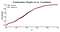Fig.1: Plot of combination weight a vs. Pearson and Spearman correlation coefficients between R1 & R3. The relationship between the correlation coefficients and a is non-linear which is evidenced by the fact that the line is not straight. Also, not shown in the plot, the relationship does not extend to negative partial correlations. The equality of the correlation coefficients reassures us of a ‘nice’ relationship between the two distributions.

Additionally, this method does not allow the generation of populations with a partial correlation that is negative. Even worse, and no surprise to statisticians, R3 is not uniformly distributed (for 0 < a <1 the null hypothesis of the Kolmogorov-Smirnov test (KS test) against a uniform distribution is rejected):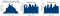Fig 2: Plot of the distributions. The distribution of R3 is not flat topped and its shape is dependent on the value of a.

The result is if the R3 distribution is used, outliers are under-represented. Depending on the purpose of the calculation, that can have a negative impact on the accuracy of the results.

What is the solution? “Obviously” use a cupola. I will get to those at later point. (Remember constraint number 1?) If a cupola solution exists that fits our constraint, as of writing this, I have yet to encounter it. In order to implement a Gaussian cupola solution in Python, one of my colleagues was doing some serious calculus and linear algebra that I hadn’t thought about since freshman year of college. Again, constraint number 1.

# Solution 2 — Seeding a Secondary Distribution

So, here is a clever idea: use R1 as the mean of a normal distribution with some σ and generate R3 from that distribution (I know I am switching from a to σ, sorry but this notation does make more sense here). Put this in a while loop so that the values of R3 are constrained on an interval. There will be some correlation between R1 & R3. The implementation of this is straightforward in most stats packages (Python being my package of choice) and is as follows:

`import scipy.stats as statsdef generate_num(center, sigma):    num = stats.norm.rvs(loc = center, scale = abs(sigma), size = 1)    while (num < 0) | (num > 1):        num = stats.norm.rvs(loc = center, scale = abs(sigma), size = 1)    if sigma > 0:        return num    else:        return 1 — num`

The results look better and there is no change in methodology to generate populations with partial negative correlation: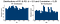Fig. 3: Distributions of R1 & R2 where R2 is generated by seeding the mean of second distribution with R1. The range of R2 is maintained with a loop that rejects values outside of the range.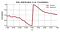Fig. 4: Relationship between the standard deviation of the secondary distribution and the correlation coefficients of R1& R2.

But there are two problems with this.

• First: how do you easily implement the while loop in Excel? If you don’t implement the filtering from the while loop you will end up with some R3 values outside of the [0,1] interval.
• Second: R3 periodically fails a KS test against a uniform distribution.

# Solution 3 — Towards a Cupola Solution

As I had returned to trying to understand cupolas, I had a fruitful conversation with a colleague. He pointed out that “linear combinations of normals are always normal.” (As written by Michael Vespe) Or, more elegantly, “the linear combination of two independent random variables having a normal distribution also has a normal distribution.” While the mathematics that go into this statement are not really basic, it resonates. Generating numerical examples is also trivial with both Python:

`import math as mtimport scipy.stats as statsrho = 0.8n1 = stats.norm.rvs(size = 1000)n2 = stats.norm.rvs(size = 1000)n3 = rho * n1 — mt.sqrt(1 — rho * * 2) * n2`

and Excel:

The Python implementation produces the following distributions:

In this case, ρ = 0.8 (another apology for the notation change, we will stick with ρ for the rest of the write up), the Pearson correlation coefficient between N1 & N3 is 0.82, and the null hypothesis of normality cannot be rejected with a KS test. Another useful property is that a normal distribution transformed by its cumulative distribution function (CDF) produces a uniform distribution.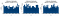Fig. 6: Uniform distributions resulting from transforming normal distributions in Fig 5. with the normal CDF

The final note — while I could not find a closed form solution on how (or why) the correlation is maintained (in general it isn’t) at 0.79, it’s pretty close. What’s nice is that this closeness holds on the interval -1<ρ <1: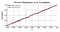Fig. 7: Relationship between r and the correlation coefficients of R1 & R3.

If all you need is the solution, STOP RIGHT HERE, we are DONE. Congratulations! But if you want to know more…

# Delving Into the Mathematics

Pushing a little further, two questions arise from this elegant solution.

• First, what is a cupola and is this a simplified version of one?
• Second, why is the linearity maintained with the square root of 1-ρ²

# Is This a Cupola?

Fast answer: yes, I think so. A cupola is the joint cumulative distribution function of uniformly distributed variables. If you look at the Wikipedia description of the Gaussian cupola, the steps can be broken down as follows: it takes two uniformly distributed variables, hits them with the normal percentage point function (each are now normally distributed), combines them, and then hits the combination with the normal cumulative distribution function returning a uniformly distributed variable.

# Why Does this Maintain the Linearity?

The mathematics on this are a bit more involved. I will not present a rigorous proof here, but hopefully some of my calculations will convince you I didn’t pluck this out of thin air.

These calculations follow the explanation of the bivariate normal distribution presented by Wolfram. So, first, the joint probability of two normally distributed variables x1 and x2 both with: μ = 0 and σ = 1 is

The bivariate normal distribution of two variables y1 and y2 is:

From the statement “the linear combination of two independent random variables having a normal distribution also has a normal distribution” we can build y₁ and y₂ as follows: y₁ = σ₁₁x₁ + σ₁₂x₂ and y₂ = σ₂₁x₁ + σ₂₂x₂. y₁ and y₂ each have mean 0 and σ₁² = σ₁₁² + σ₁₂² and σ₂² = σ₂₁² + σ₂₂² with covariance matrix

The relationship between X = [x₁,x₂] and Y = [y₁,y₂] can be expressed in matrix form, allowing us to elegantly calculate the x’s as a function of the y’s:

Now, if we choose σ₁₁ = 1, σ₁₂ = 0 and σ₂₁ = ρ and the off-diagonal terms in the covariance matrix are ρσ₁σ₂, it’s required that σ₂₂ is the square root of 1-ρ². Also, when we work through the math, we get:

So, not a proof, but some confidence building calculations.

# Conclusion

We have generated two uniformly distributed partially correlated random distributions. The solution is demonstrated in both a scripting language and Excel. The partially correlated uniform distributions can be transformed by the percentage point function of any other distribution thus extending this solution to an arbitrary set of distributions. Finally, the mathematical arguments, while somewhat complex, do resonate even without fully working through the calculations. This solution can be implemented across tool sets and the explanation is accessible to the Business, conforming to both our stated criteria.

DISCLOSURE STATEMENT: These opinions are those of the author. Unless noted otherwise in this post, Capital One is not affiliated with, nor is it endorsed by, any of the companies mentioned. All trademarks and other intellectual property used or displayed are the ownership of their respective owners. This article is © 2018 Capital One.

## Capital One Tech

#### The low down on our high tech from the engineering experts at Capital One. Learn about the solutions, ideas and stories driving our tech transformation.

Welcome to a place where words matter. On Medium, smart voices and original ideas take center stage - with no ads in sight. Watch
Follow all the topics you care about, and we’ll deliver the best stories for you to your homepage and inbox. Explore
Get unlimited access to the best stories on Medium — and support writers while you’re at it. Just \$5/month. Upgrade